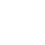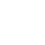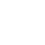Cms技巧

# Phpcms V9设置文章定时发布程序代码

phpcms v9 本身是没有定时发布这一项功能的，这需要我们进行二次开发才能实现，关于定时发布的好处不用多说了吧，当我们在没有时间进行更新站点的时候，不会出现文章断更的情况，比如过年或者自己过节没有时间更新了，可以提前写好文章，让其自动进行更新，在 wordpress 中有这样一个很好的时间机制，下面夏日博客就来说下在 phpcms v9 中如何实现文章定时的发布，这是在 pc 论坛上面看到的一个方法，感觉不错就分享下代码吧。

1、修改api/count.php这一文件，在PHP语句结束代码 ?>前，加入以下代码：

\$modelid = \$modelid ? \$modelid : intval(\$_GET['modelid']);
\$content_db = \$content_db ? \$content_db : pc_base::load_model('content_model');
\$content_db->set_model(\$modelid);
\$where = ' status = 1 and inputtime <= '.SYS_TIME;
\$r = \$content_db->count(\$where);
if( !empty(\$r) ){ //执行update操作
\$ids = \$content_db->select(\$where, 'id,catid', \$r, '', '', 'id');
foreach(\$ids AS \$kid=>\$v){
\$catid = \$v['catid'];
\$id = \$kid;
\$r = \$content_db->get_content(\$catid,\$id);
\$urls = \$urlobj->show(\$id, 0, \$catid, \$r['inputtime'], \$r['prefix'],\$r,'add');
if(\$urls['content_ishtml']) \$html->show(\$urls,\$urls['data'],0);
\$html->index();
\$html->create_relation_html(\$catid);
}
\$content_db->update( array('status'=>99),\$where );
}

2、在文章页面的模板里边添加统计代码：

TOPQQ客服18910140161